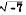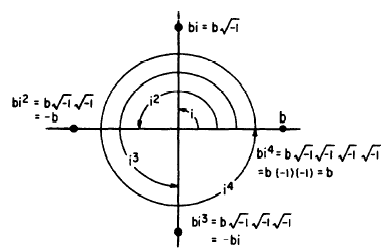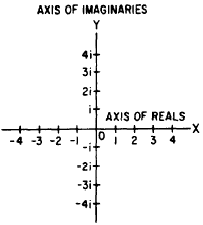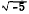The complex planeCustom SearchTHE COMPLEX PLANE All imaginary numbers may be represented graphically along a line extending through zero and perpendicular to the line representing the real numbers. This line may be considered in- finite in both the positive and negative directions, and all multiples of i may be represented on it. This graph is similar to the rectangular coordinate system studied earlier. In this system, the vertical or y axis is called the axis of imaginaries, and the horizontal or x axis is called the axis of reals. In the rectangular coordinate system, real numbers are laid off on both the x and y axes and the plane on which the axes lie is called the real plane. When the y axis is the axis of imaginaries, the plane determined by the x and y axes is called the COMPLEX PLANE (fig. 15-6). In any system of numbers a unit is necessary for counting. Along the real axis, the unit is the number 1. As shown in figure 15-6, along the imaginary axis the unit is i. Numbers that lie along the imaginary axis are called PURE IMAGINARIES. They will always be some multiple of i, the imaginary unit. The numbers 5i, 3i, andare examples of pure imaginaries. NUMBERS IN THE COMPLEX PLANE All numbers in the complex plane are complex numbers, including reals and pure imaginaries. However, since the reals and imaginaries haveFigure 15-5.-Operation with powers of i.Figure 15-6. -The complex plane. the special property of being located on the axes, they are usually identified by their distinguishing names. The term complex number has been defined as the indicated sum or difference of a real number and an imaginary number. For example, 3 + 5or 3 + 5i, 2 - 6i, and -2 +are complex numbers. In the complex number 7 - i, 7 is the real part and -1the imaginary part. All complex numbers correspond to the general form a + bi, where a and b are real numbers. When a has the value 0, the real term disappears and the complex number becomes a pure imaginary. When b has the value of 0, the imaginary term disappears and the complex number becomes a real number. Thus, 4 may be thought of as 4 + 0i, and 3i may be considered 0 + 3i. From this we may reason that the real number and the pure imaginary number are special cases of the complex number. Consequently, the complex number may be thought of as the most general form of a number and can be construed to include all the numbers of algebra as .shown in the chart in Figure 15-7.Integrated Publishing, Inc. - A (SDVOSB) Service Disabled Veteran Owned Small Business